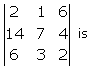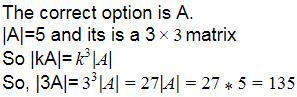# Test: Properties And Applications Of Determinants

## 5 Questions MCQ Test Mathematics (Maths) Class 12 | Test: Properties And Applications Of Determinants

Description
This mock test of Test: Properties And Applications Of Determinants for JEE helps you for every JEE entrance exam. This contains 5 Multiple Choice Questions for JEE Test: Properties And Applications Of Determinants (mcq) to study with solutions a complete question bank. The solved questions answers in this Test: Properties And Applications Of Determinants quiz give you a good mix of easy questions and tough questions. JEE students definitely take this Test: Properties And Applications Of Determinants exercise for a better result in the exam. You can find other Test: Properties And Applications Of Determinants extra questions, long questions & short questions for JEE on EduRev as well by searching above.
QUESTION: 1

### Points (a,a,c),(1,0,1) and (c,c,b) are collinear if​

Solution:

a(-c) - a(b-c) + c(c) = 0
- ac - ab + ac + c2 = 0
c2 = ab

QUESTION: 2

### If A is a square matrix of order 3 and |A| = 7 then |AT| =​

Solution:

The determinant of a square matrix is the same as the determinant of its transpose.

QUESTION: 3

### The value of determinant​

Solution:
QUESTION: 4

If A is a square matrix of order 3 and |A| = 5 then |3A| = ?​

Solution:QUESTION: 5

If A is square matrix of order 3 and |A| = 7 then |AT| = ______​

Solution:

The determinant of a square matrix is the same as the determinant of its transpose. Therefore |A’| = 7